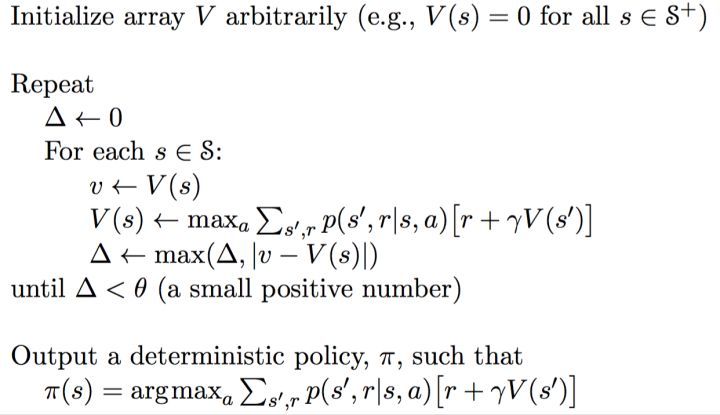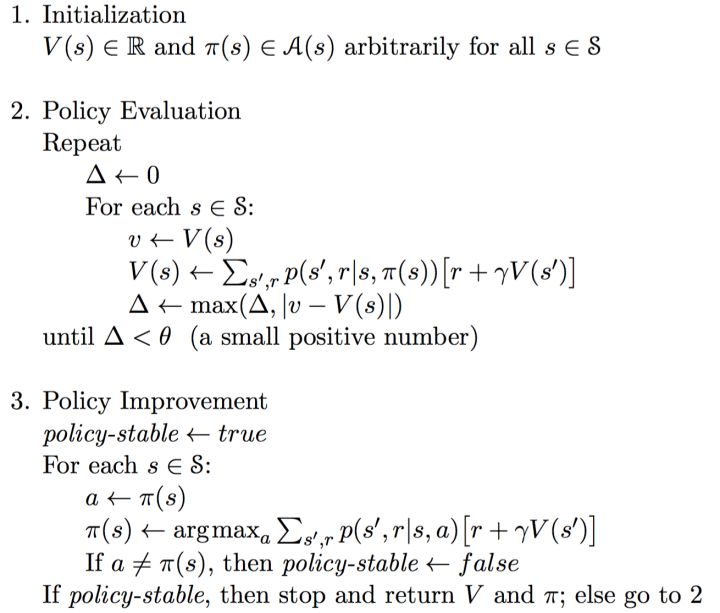## 强化学习的任务$(s_1,a_1,r_1,s_2,a_2,r_2,…s_t,a_t,r_t)$

## MDP（Markov Decision Process）

1. $S$ 是一个有限状态集
2. $A$ 是一个有限动作集
3. $P$ 是一个状态转移概率矩阵，$P_a(s, s′)=P(s_{t+1}=s′|s_t=s, a_t=a)$ 表示在状态 $s$ 下执行动作 $a$ 后转移到状态 $s′$ 的概率
4. $R$ 是一个奖励函数，$R_a(s, s′)$ 表示在状态 $s$ 下执行动作 $a$ 后转移到状态 $s′$ 所得到的即时回报(reward)
5. $\gamma$ 是一个折扣因子,一般取值在 [0,1]; 用来区分当前回报和未来回报的重要性，一般会加在未来的回报前，减小未来回报的权重。

## 回报与价值函数

$G_t = R_{t+1} + \gamma R_{t+2} + … = \sum_{k=0}^\infty\gamma^kR_{t+k+1}$

$V(s) = \mathbb E[G_t|S_t = s]$

1. 直接优化策略 $\pi(a|s)$ 或者 $a = \pi(s)$ 使得回报更高
2. 通过估计 value function 来间接获得优化的策略。道理很简单，通过价值函数可以知道每一种状态的好坏，这样我们就知道该怎么选择了（如选择动作使得下一状态的潜在价值最大），而这种选择就是我们想要的策略。

## Bellman方程

\begin{align} V(s) & = \mathbb E[G_t|S_t = s]\\ & = \mathbb E[R_{t+1}+\gamma R_{t+2} + \gamma ^2R_{t+3} + …|S_t = s]\\ & = \mathbb E[R_{t+1}+\gamma (R_{t+2} + \gamma R_{t+3} + …)|S_t = s]\\ & = \mathbb E[R_{t+1}+\gamma G_{t+1}|S_t = s]\\ & = \mathbb E[R_{t+1}+\gamma v(S_{t+1})|S_t = s] \end{align}

$$V(s) = \mathbb E[R_{t+1} + \gamma V(S_{t+1})|S_t = s]$$

## 从 MDP 到 Reinforcement Learning

$$\pi(s):=\arg \max_a\ {\sum_{s’}P_{a}(s,s’)(R_{a}(s,s’)+\gamma V(s’))}$$

$$V(s) := \sum_{s’}P_{\pi(s)}(s,s’)(R_{\pi(s)}(s,s’) + \gamma V(s’))$$

### Value iteration

$$V_{i+1}(s) := \max_a \sum_{s’} P_a(s,s’)(R_a(s,s’) + \gamma V_i(s’))$$### Policy iteration

Policy iteration同时更新价值 $V$ 和策略 $\pi$, 且一般可分成两步：

1. Policy Evaluation，策略评估，就是上面公式2的过程。目的是在策略固定的情况下更新Value Function 直到 value 收敛，从另一个角度来讲就是为了更好地估计基于当前策略的价值
2. Policy Improvement，策略改进，就是上面公式1的过程。就是根据更新后的 Value Function 来更新每个状态下的策略直到策略稳定### 区别与局限

Q-learning 实际上就是采用这种思想的，Q-Learning的基本思想是根据 value iteration 得到的，但要明确一点是 value iteration 每次都对所有的Q值更新一遍，也就是所有的状态和动作。但事实上在实际情况下我们没办法遍历所有的状态，还有所有的动作，因此，我们只能得到有限的系列样本。具体的算法流程会再下一篇文章具体介绍。